# Runes Power-Up Success Rate and Mana to +15

Welcome to the Guide for Runes Power-Up Success Rate and Mana Expenditure to +3, +6, +9, +12 and +15.

In Beathem, we performed a detailed analysis using 1800 iterations in order to determine the chances of success when powering up runes of different grades. The results will allow to Summoners War gamers to understand better the effort needed for powering up runes up to +15.

## Pre-Information

All the calculations displayed below are based in a statistical analysis and is attached to statistical errors based in the sample of the data, and therefore, confidence intervals will determine the quality of the analysis. The following points are important to be considered:

• Analysis includes different runes grades (3*, 4*, 5* and 6*), but low grade runes are not included (1* and 2*).
• The sample of the data is an important factor, and therefore the numbers calculated below are only an approximation. Even if the data is 100% reliable, it doesn’t mean that the outputs are gonna perform equal for each player. Summoners War is a RNG based game and therefore luck and/or probability will be always a factor to be considered.
• The results will be updated every time we will gather 1000 new iterations.
• Rare, Hero and Legend runes have been considered to not influence in the success rate.

## What will you find in this guide?

• Probability of Success when powering-up runes.
• Average Mana Cost for power-up a rune up to certain level (total, fractioned and in intervals of 3).
• Data sample size used for each probability.
• Total Mana used to perform the analysis.

## What will you not find in this guide?

In this guide you will not find the types of runes or the performance of each of them. You will not find if it worth to upgrade runes to +15 for different grades because this is only based in the player own criteria.

This guide is deterministic, quantitative and objective and does not include any personal opinions or thoughts. Thus, can be used as a reference point for any further guide or more complex analysis.

## Probability of Success when Powering-Up Runes

The table below shows the probability of success when powering-up runes (experiment has been performed without using power-up stones). Note that 1* and 2* runes are not included due to the low performance of these runes.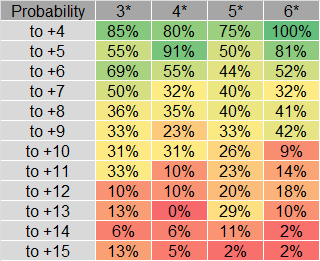Table with the sample of the data is included. On this way, the reader can have an idea of the scope of the analysis and decide by his/her own criteria if the probability is close enough to a real case.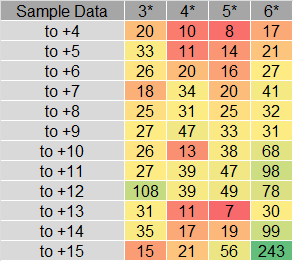It is easy to realise that for cases like “Probability to +13” for “4* Rune” the probability is 0%, meaning that the sample of the data is not big enough to give a result with acceptable confidence intervals, and therefore, more data need to be gathered for further analysis.

## Average Mana Cost

In order to determine the Average Mana Cost based in the previous table results, it is needed to include the mana cost for powering-up runes (see below).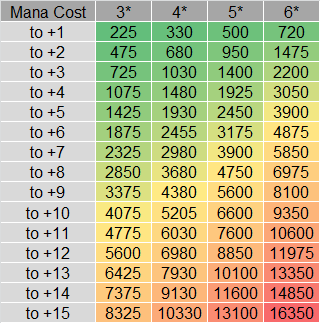The next step is to calculate the average mana needed. In Beathem, we believe that the best way of present these results is dividing in two groups:

• Average Mana needed starting from +0 (first table).
• Average Mana needed fractioned in groups of 3 levels (second table).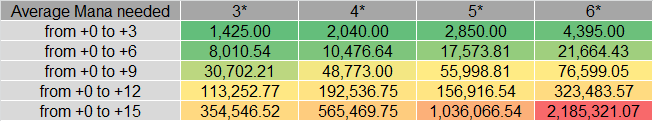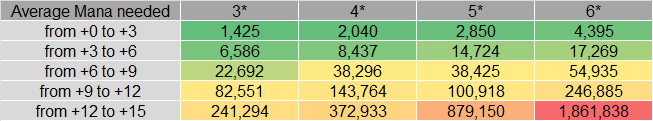The equations used in these mana predictions are very simple and consider the mana cost and the probability of success.

For example:

“Average Mana needed from +3 to +6” = [(Mana Cost to +4) / (Probability to +4)][(Mana Cost to +5) / (Probability to +5)][(Mana Cost to +4) / (Probability to +5)]

Applying the equation for 3*  runes we get the following:

“Average Mana needed from +3 to +6” = (1075 / 0.85) + (1425 /0.55) + (1875 / 0.69) = 6,586 Mana

## Data Sample Size and Total Mana Used for Analysis

The data sample size is included in the table below. A total of 1801 iterations have been performed. The sample size is intended to be increased and updated as more iterations are performed.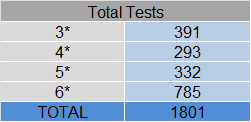The total mana used in order to perform the previous analysis is included as well in the table below.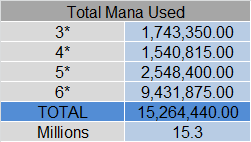A total of 15,264,000 mana has been used for this analysis. On this way is easier to see the effort performed for the develop of this Summoners War Guide.

## Future Work

• Include more iterations in order to reduce the confidence intervals in the predictions.
• Include 1* and 2* runes for the use of new Summoners War players.
• Include the rarity of the rune and perform an analysis to see if it affects to the success rate.

This guide finish here. We hope the above information is useful and can be applied for as many players as possible.

* If you have any comment or input for this guide base we encourage you to leave a comment in the comment box below.